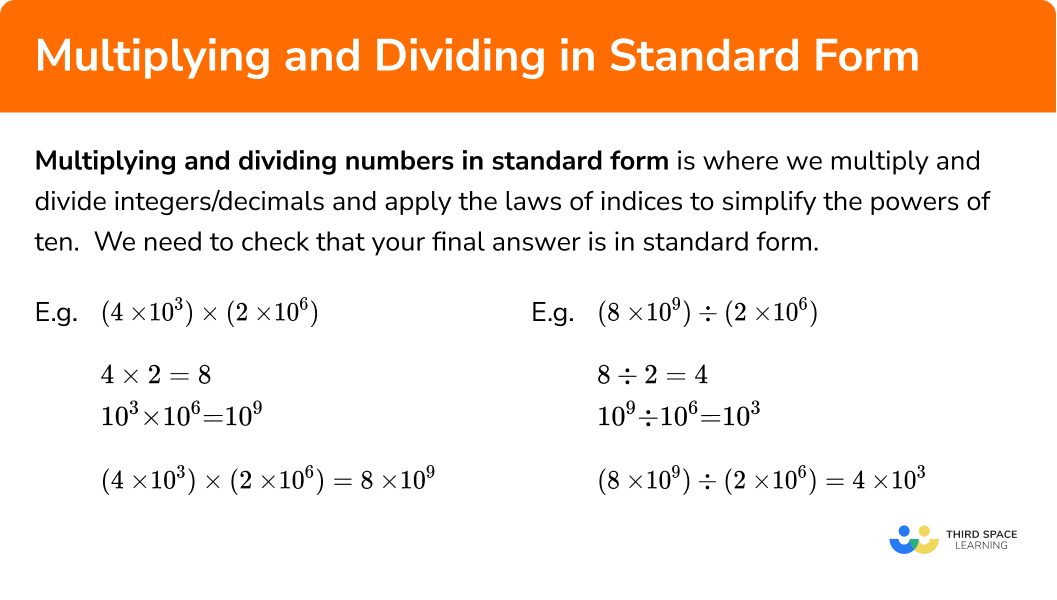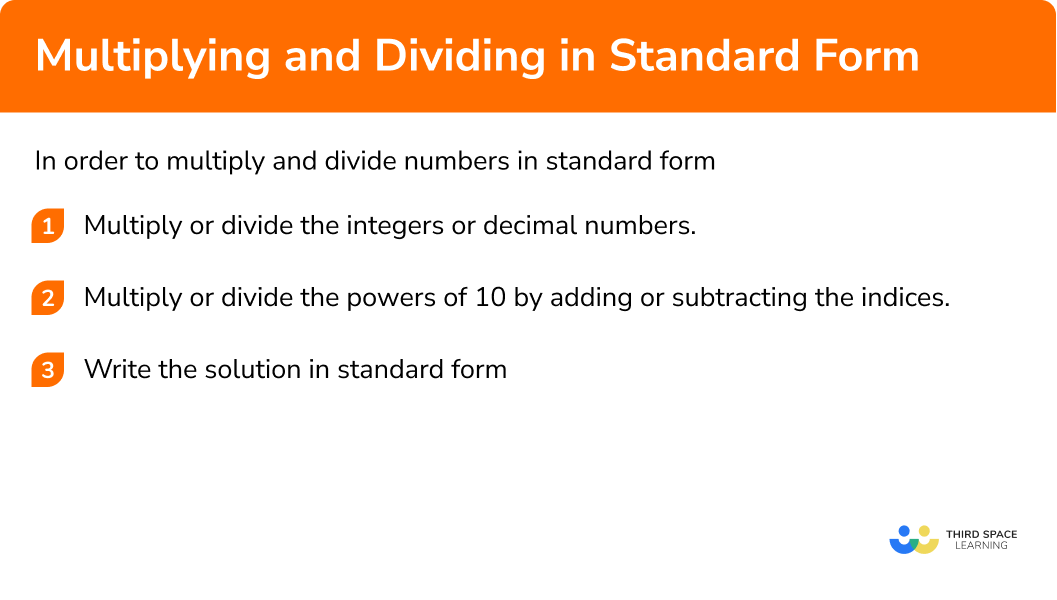GCSE Maths Number Standard Form

Multiplying and Dividing in Standard Form

# Multiplying and dividing in standard form

Here we will learn about multiplying and dividing numbers in standard form including how to multiply, divide and square numbers in standard form.

There are also multiplying and dividing numbers in standard form worksheets based on Edexcel, AQA and OCR exam questions, along with further guidance on where to go next if you’re still stuck.

## What is multiplying and dividing in standard form?

Multiplying and dividing numbers in standard form is where we multiply and divide integers and decimals and apply the laws of indices to simplify the powers of ten. You also need to check that your final answer is in standard form.

E.g.

$(4\times10^{3})\times(2\times10^{6})$

$4\times2 = 8$

$10^{3}\times10^{6}\quad=\quad10^{9}$

$(4\times10^{3})\times(2\times10^{6})\quad=\quad8\times10^{9}$

E.g.

$(8\times10^{9})\quad \div \quad(2\times10^{6})$

$8 \div2 = 4$

$10^{9}\quad \div \quad10^{6}\quad=\quad10^{3}$

$(8\times10^{9})\quad \div \quad(2\times10^{6})\quad=\quad4\times10^{3}$

There are many applications of multiplying and dividing standard form numbers which includes applying it to area, volume, surface area, equations and Pythagoras’ theorem.

### What is multiplying and dividing in standard form?## How to multiply and divide in standard form

In order to multiply and divide numbers in standard form:

1. Multiply or divide the integers or decimal numbers.
2. Multiply or divide the powers of ten by adding or subtracting the indices.
3. Write the solution in standard form, checking that the first part of the number is \pmb{1\leq{x}\lt10}.

### How to multiply and divide with standard form## Multiplying and dividing standard form examples

### Example 1: multiplying numbers in standard form

Work out:

$(3 \times 10^{4}) \times (2 \times 10^{5})$

1. Multiply or divide the integers or decimal numbers.

$3 \times 2 = 6$

2Multiply or divide the powers of ten by adding or subtracting the indices.

$10^{4} \times 10^{5} = 10^{9}$

3Write the solution in standard form, checking that the first part of the number is \pmb{1\leq{x}\lt10}.

$6 \times 10^{9}$

This number is already in standard form.

### Example 2: multiplying numbers in standard form

Work out:

$(3 \times 10^{5}) \times (6 \times 10^{-2})$
$3\times6 = 18$

$10^{5} \times 10^{-2}=10^{3}$

$18 \times 10^{3}$

18 is greater than 10 so divide 18 by 10 which gives the result 1.8.

To compensate, you need to multiply the power of ten by 10 which has the effect of adding one to the power, which gives the result 10^{4} .

The final result is 1.8 \times 10^{4}.

### Example 3: dividing numbers in standard form

Work out:

$(8 \times 10^{7}) \div (2 \times 10^{5})$
$8\div2 = 4$

$10^{7} \div 10^{5} = 10^{2}$

$4 \times 10^{2}$

This number is already in standard form.

### Example 4: dividing numbers in standard form

Calculate:

$(3 \times 10^{4}) \div (6 \times 10^{-3})$

$3\div6 = 0.5$

$10^{4} \div 10^{-3} = 10^{7}$

$0.5\times10^{7}$

0.5 is less than 1 so multiply 0.5 by 10 which gives the result 5.

To compensate, you need to divide the power of ten by 10 which has the effect of subtracting one from the power, which gives the result 10^{6}.

The final result is 5\times10^{6}.

### Example 5: squaring numbers in standard form

Calculate:

$(8 \times 10^{4})^{2}$

We know that

$\left(8 \times 10^{4}\right)^{2}=\left(8 \times 10^{4}\right) \times\left(8 \times 10^{4}\right)$

Let’s first work out 8\times8 = 64

Simplify 10^{4} \times 10^{4} = 10^{8}

$64 \times 10^{8}$

64 is greater than 10 so divide 64 by 10 which gives the result 6.4.

To compensate, you need to multiply the power of ten by 10 which has the effect of adding one to the power, which gives the result 10^{9}.

The final result is 6.4 \times 10^{9}.

### Example 6: cubing numbers in standard form

Calculate:

$(6 \times 10^{4})^{3}$

We know that (6\times10^{4})^{3}= (6\times10^{4})\times(6\times10^{4}) \times (6\times10^{4})

Let’s first work out 6\times6\times6 = 216

Simplify 10^{4} \times 10^{4} \times 10^{4} = 10^{12}

$216 \times 10^{12}$

216 is greater than 10 so divide 216 by 100 which gives the result 2.16.

To compensate, you need to multiply the power of ten by 100 which has the effect of adding 2 to the power, which gives the result 10^{14}.

The final result is 2.16 \times 10^{14}.

### Common misconceptions

• Multiplying the powers

When multiplying numbers in standard form, a common mistake is multiplying the powers rather than adding the powers.

E.g.

(4\times10^{5})\times(2\times10^{3}) = 8\times10^{8} .
The power of ten is 10^{8} , NOT 10^{15}.

• Dividing the powers

When dividing numbers in standard form, a common mistake is dividing the powers rather than subtracting the powers.

E.g.

(8\times10^{9})\div(2\times10^{3}) = 4\times10^{6}
The power of ten is 10^{6} , NOT 10^{3}.

• Not converting solutions to standard form

After calculating with standard form, a common mistake is not checking that the first part of the number is 1 x < 10.

E.g.

45 x 10^{6} is not in standard form.

• Negative numbers

Not adding or subtracting negative powers correctly, especially when dividing by a negative power as here you would be subtracting a negative number which has the same effect as adding.

Multiplying and dividing standard form is part of our series of lessons to support revision on standard form. You may find it helpful to start with the main standard form lesson for a summary of what to expect, or use the step by step guides below for further detail on individual topics. Other lessons in this series include:

### Practice multiplying and dividing standard form questions

5 \times 10^{10}6 \times 10^{10}6 \times 10^{24}5 \times 10^{24}2 \times 3 = 6
10^{6} \times 10^{4} = 10^{10}
(2 \times 10^{6}) \times (3 \times 10^{4}) = 6 \times 10^{10}

28 \times 10^{11}2.8 \times 10^{11}2.8 \times 10^{12}0.28 \times 10^{13}4 \times 7 = 28\\ 10^{5} \times 10^{6} = 10^{11}\\ (4 \times 10^{5}) \times (7 \times 10^{6}) = 28 \times 10^{11}

This answer is not in standard form because 28 is not between 1 and 10 . We need to divide 28 by 10 and, to compensate, multiply 10^{11} by 10 , increasing the power by 1 .

This gives us 2.8 \times 10^{12}

3. Work out (6\times10^{8}) \div (3\times10^{5}) . Write your answer in standard form.

2 \times 10^{3}2 \times 10^{1.6}3 \times 10^{3}0.2 \times 10^{4}6 \div 3 = 2\\ 10^{8} \div 10^{5} = 10^{3}\\ (6 \times 10^{8}) \div (3 \times 10^{5}) = 2 \times 10^{3}

4. Work out (2\times10^{4})\div (8\times10^{-3}) . Write your answer in standard form.

4 \times 10^{7}0.25 \times 10^{7}2.5 \times 10^{6}2.5 \times 10^{8}2 \div 8 = 0.25\\ 10^{4} \div 10^{-3} = 10^{7}\\ (2 \times 10^{4}) \div (8 \times 10^{-3}) = 0.25 \times 10^{7}

This answer is not in standard form because 0.25 is not between 1 and 10 . We need to multiply 0.25 by 10 and, to compensate, divide 10^{7} by 10 , decreasing the power by 1 .

This gives us 2.5 \times 10^{6}

25 \times 10^{6}25 \times 10^{5}1.0 \times 10^{7}2.5 \times 10^{7}(5 \times 10^{3})^{2}=(5 \times 10^{3}) \times (5 \times 10^{3})\\ 5 \times 5 = 25\\ 10^{3} \times 10^{3}=10^{6}\\ (5 \times 10^{3})^{2}=25 \times 10^{6}

This answer is not in standard form because 25 is not between 1 and 10 . We need to divide 25 by 10 and, to compensate, multiply 10^{6} by 10 , increasing the power by 1 .

This gives us 2.5 \times 10^{7}

4.9 \times 10^{11}3.43 \times 10^{17}343 \times 10^{15}3.43 \times 10^{127}(7 \times 10^{5})^{3}=(7 \times 10^{5}) \times (7 \times 10^{5}) \times (7 \times 10^{5})\\ 7 \times 7 \times 7 = 343\\ 10^{5} \times 10^{5} \times 10^{5} =10^{15}\\ (7 \times 10^{5})^{3}=343 \times 10^{15}

This answer is not in standard form because 343 is not between 1 and 10 . We need to divide 343 by 100 and, to compensate, multiply 10^{15} by 100 , increasing the power by 2 .

This gives us 3.43 \times 10^{17}

### Multiplying and dividing standard form GCSE questions

1. A cube has side lengths of 2\times10^{3} metres. Calculate the surface area of the cube. Write your answer in standard form.

(3 marks)

Finding the area of one face: 4\times10^{6}

(1)

Multiply by 6 or correct answer not in standard form.

(1)

2.4\times10^{7}m^{2}

(1)

(2 marks)

9.3\div 3.1 = 3 or 10^{8} \div 10^{2} = 10^{6}

(1)

3\times10^{6}

(1)

3.

a) Write 75000 in standard form.

b) Write 0.03 in standard form.

c) Using your answers to a) and b), and also the formula F=ma, calculate the value of F when m=75000 and a=0.03. Write your answer in standard form.

(5 marks)

a) 75000 in standard form is 7.5 \times 10^{4}

(1)

b) 0.03 in standard form is 3 \times 10^{-2}

(1)

c) F = ma

Therefore,

F=7.5 \times 10^{4} \times 3 \times 10^{-2}

(1)

7.5 \times 3 =22.5

10^{4} \times 10^{-2} = 10^{2}

(1)

22.5 \times 10^{2} = 2.25 \times 10^{3}

F=2.25 \times 10^{3}

(1)

## Learning checklist

You have now learned how to:

• Multiply numbers in standard form
• Divide numbers in standard form
• Square and cube numbers in standard form

## Still stuck?

Prepare your KS4 students for maths GCSEs success with Third Space Learning. Weekly online one to one GCSE maths revision lessons delivered by expert maths tutors.

Find out more about our GCSE maths tuition programme.